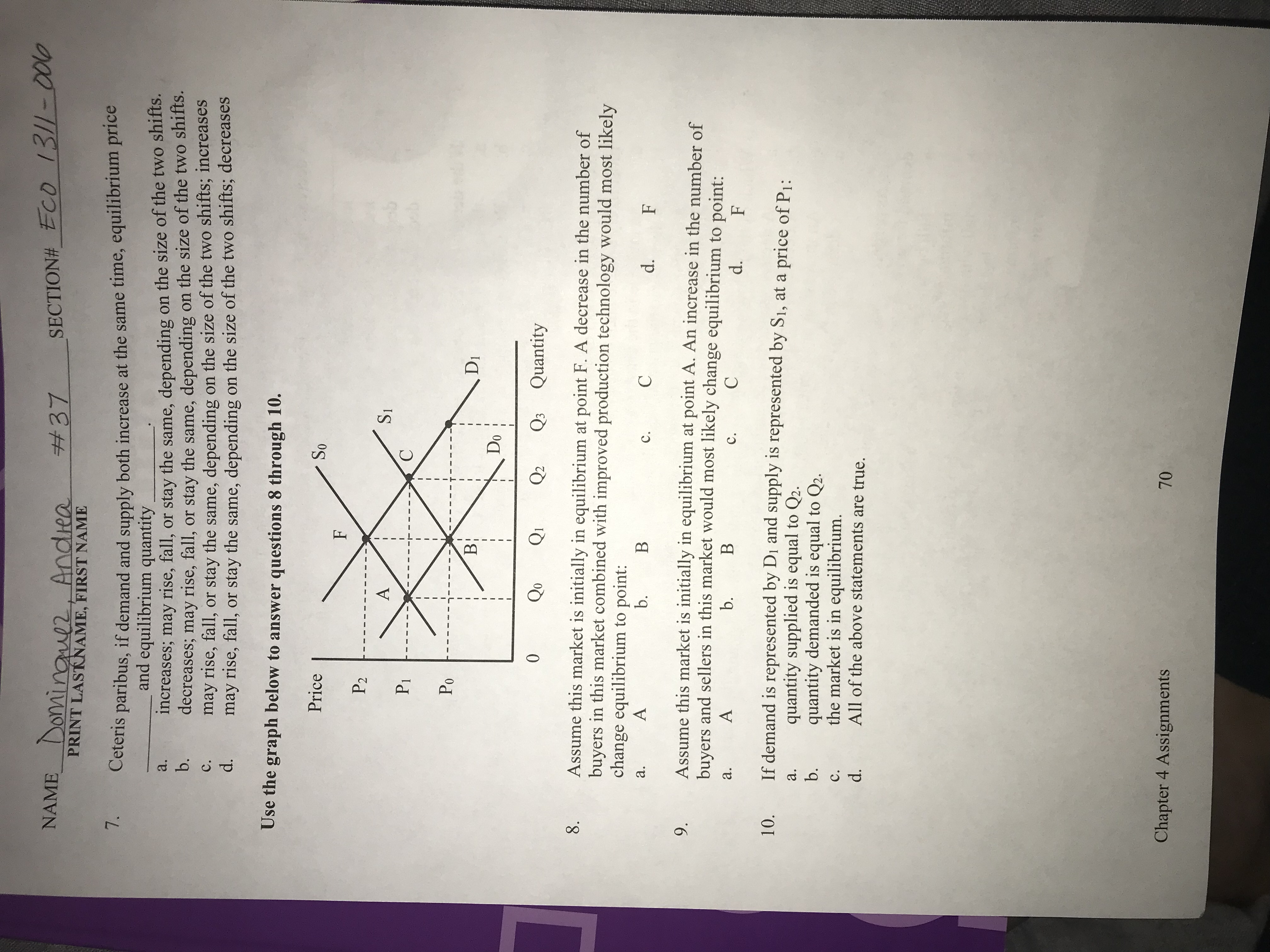# NAME Domingul2, AndieaSECTION#_ECO 13/1-0010#37PRINT LASTKNAME, FIRST NAME7.Ceteris paribus, if demand and supply both increase at the same time, equilibrium priceand equilibrium quantityincreases; may rise, fall, or stay the same, dependingdecreases; may rise, fall,may rise, fall,may rise, fall, or stay the same, dependingon the size of the two shifts.on the size of the two shifts.a.b.or stay the same, dependingor stay the same, depending on the size of the two shifts; increaseson the size of the two shifts; decreasesс.d.Use the graph below to answer questions 8 through 10.PriceSoFP2ASIPiСPoВ:DIDo0QoQ2Q3 Quantity8.Assume this market is initially in equilibrium at point F. A decrease in the number ofbuyers in this market combined with improved production technology would most likelychange equilibrium to point:Аb.Ва.Cс.d.FAssume this market is initially in equilibrium at point A. An increase in the number ofbuyers and sellers in this market would most likely change equilibrium to point:9.Аb.Ва.Сс.d.FIf demand is represented by Di and supply is represented by S1, at a price of P1:10.quantity supplied is equal to Q2.quantity demanded is equal to Q2.the market is in equilibrium.All of the above statements are true.а.b.с.d.Chapter 4 Assignments70

Question
3 views

I need help with question 7.help_outlineImage TranscriptioncloseNAME Domingul2, Andiea SECTION#_ECO 13/1-0010 #37 PRINT LASTKNAME, FIRST NAME 7. Ceteris paribus, if demand and supply both increase at the same time, equilibrium price and equilibrium quantity increases; may rise, fall, or stay the same, depending decreases; may rise, fall, may rise, fall, may rise, fall, or stay the same, depending on the size of the two shifts. on the size of the two shifts. a. b. or stay the same, depending or stay the same, depending on the size of the two shifts; increases on the size of the two shifts; decreases с. d. Use the graph below to answer questions 8 through 10. Price So F P2 A SI Pi С Po В: DI Do 0 Qo Q2 Q3 Quantity 8. Assume this market is initially in equilibrium at point F. A decrease in the number of buyers in this market combined with improved production technology would most likely change equilibrium to point: А b. В а. C с. d. F Assume this market is initially in equilibrium at point A. An increase in the number of buyers and sellers in this market would most likely change equilibrium to point: 9. А b. В а. С с. d. F If demand is represented by Di and supply is represented by S1, at a price of P1: 10. quantity supplied is equal to Q2. quantity demanded is equal to Q2. the market is in equilibrium. All of the above statements are true. а. b. с. d. Chapter 4 Assignments 70 fullscreen
check_circle

Step 1

The correct option for the above-men...

### Want to see the full answer?

See Solution

#### Want to see this answer and more?

Solutions are written by subject experts who are available 24/7. Questions are typically answered within 1 hour.*

See Solution
*Response times may vary by subject and question.
Tagged in